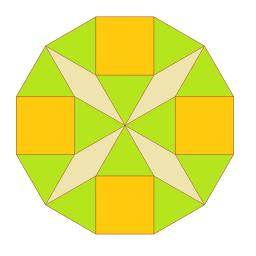# Cuboid

The volume of the cuboid is 245 cm3. Each cuboid edge length can be expressed by a integer greater than 1 cm. What is the surface area of the cuboid?

Correct result:

S =  238 cm2

#### Solution:We would be pleased if you find an error in the word problem, spelling mistakes, or inaccuracies and send it to us. Thank you!Tips to related online calculators
Do you want to convert length units?
Tip: Our volume units converter will help you with the conversion of volume units.

## Next similar math problems:

• Minimum surfaceFind the length, breadth, and height of the cuboid shaped box with a minimum surface area, into which 50 cuboid shaped blocks, each with length, breadth and height equal to 4 cm, 3 cm and 2 cm respectively can be packed.
• Identical cubesFrom the smallest number of identical cubes whose edge length is expressed by a natural number, can we build a block with dimensions 12dm x 16dm x 20dm?
• Cuboid wallsCalculate the volume of the cuboid if its different walls have area of 195cm², 135cm² and 117cm².
• CremonsThe freight wagon is shaped like cuboid 21m and 3.5m and 4.2m How many cremons can be loaded if one is a cube with an edge length of 7 cm?
• RectangleThe perimeter of the rectangle is 22 cm and content area 30 cm2. Determine its dimensions, if the length of the sides of the rectangle in centimeters is expressed by integers.
• Cuboid edgesCalculate the volume and surface of a cuboid whose edge lengths are in the ratio 2: 3: 4 and the longest edge measures 10cm.
• Tetrahedral prismThe height of a regular tetrahedral prism is three times greater than the length of the base edge. Calculate the length of the base edge, if you know that the prism volume is 2187 cm3.
• Prism XThe prism with the edges of the lengths x cm, 2x cm and 3x cm has volume 20250 cm3. What is the area of surface of the prism?
• Bricks pyramidHow many 50cm x 32cm x 30cm brick needed to built a 272m x 272m x 278m pyramid?
• ContainerThe container-shaped box with internal dimensions of 3.9 m, 3.25 m and 2.6 m was completely filled with goods in the same cubic boxes. How long edge could this box have?
• Cuboid - edgesThe cuboid has dimensions in ratio 4: 3: 5, the shortest edge is 12 cm long. Find: (A) the lengths of the remaining edges, (B) the surface of the cuboid, (C) the volume of the cuboid
• Two rectangular boxesTwo rectangular boxes with dimensions of 5 cm, 8 cm, 10 cm, and 5 cm, 12 cm, 1 dm are to be replaced by a single cube box of the same cubic volume. Calculate its surface.
• Cube-shaped boxDesign the size of the smallest possible cube-shaped box where three types of 3cm, 5cm, 6cm small cubes could be stacked to make full use of the box space (each type of cube separately). Can you find out how many smallest cubes are in the box?
• Surface of cubesPeter molded a cuboid 2 cm, 4cm, 9cm of plasticine. Then the plasticine split into two parts in a ratio 1:8. From each part made a cube. In what ratio are the surfaces of these cubes?
• Length of the edgeFind the length of the edge of a cube that has a cm2 surface and a volume in cm3 expressed by the same number.
• SegmentsLine segments 62 cm and 2.2 dm long we divide into equal parts which lengths in centimeters is expressed integer. How many ways can we divide?
• Copper plateCalculate the thickness of the copper plate with a density 8.7 g/cm³ measuring 1.5 meters and 80 cm and its weight is 3.65 kg